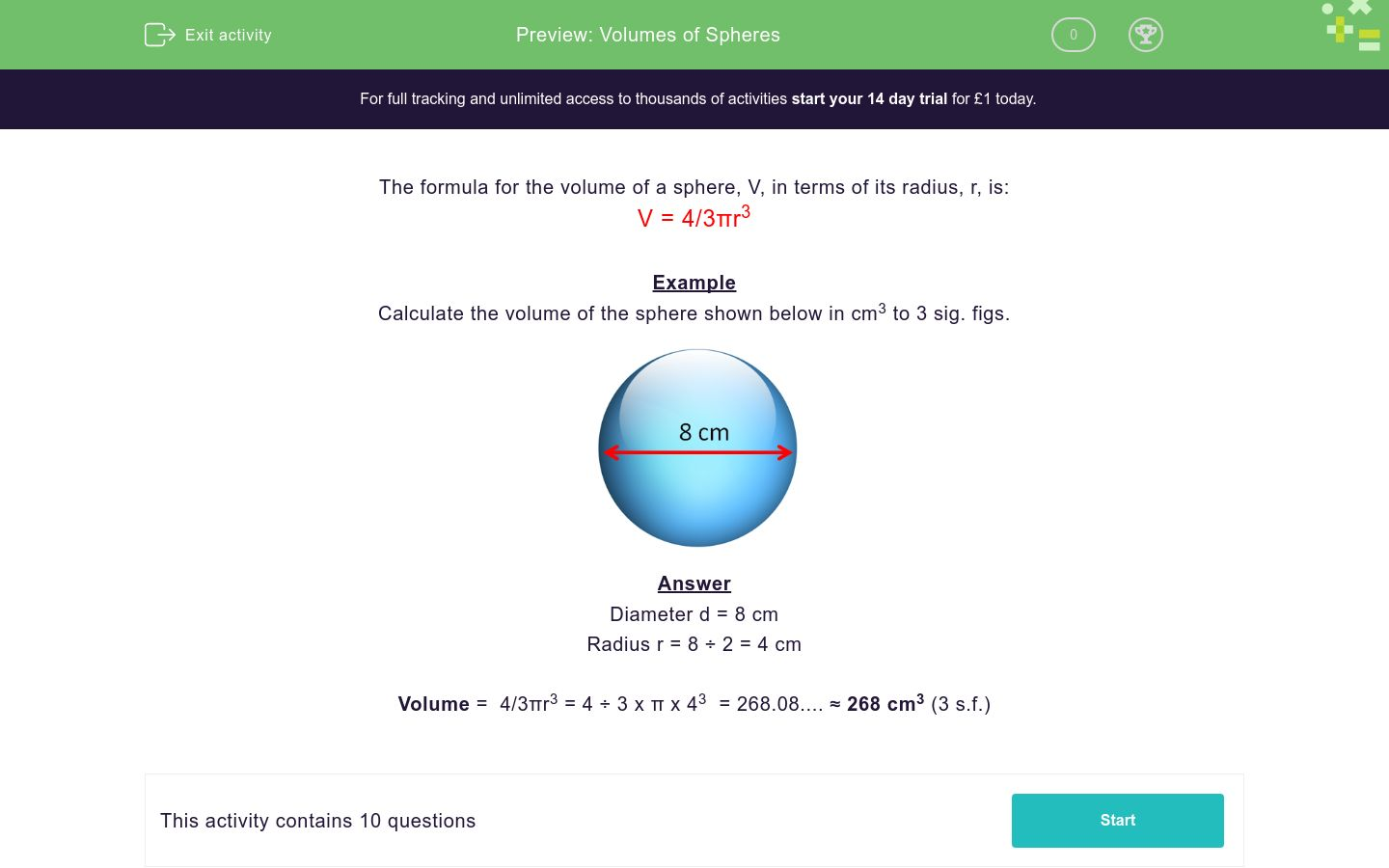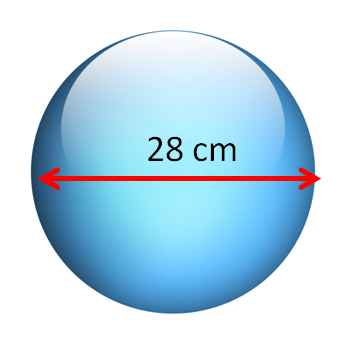# Volumes of Spheres

In this worksheet, children must calculate the volume of spheres, given their radii or diameters.Key stage:  KS 3

Curriculum topic:   Geometry and Measures

Curriculum subtopic:   Use 3D Shape Knowledge to Solve Problems

Difficulty level:### QUESTION 1 of 10

The formula for the volume of a sphere, V, in terms of its radius, r, is:

V = 4/3πr3

Example

Calculate the volume of the sphere shown below in cm3 to 3 sig. figs.Diameter d = 8 cm

Radius r = 8 ÷ 2 = 4 cm

Volume =  4/3πr3 = 4 ÷ 3 x π x 43  = 268.08.... ≈ 268 cm3 (3 s.f.)

Calculate the volume of the sphere shown below in cm3 to 3 sig. figs.

(just write the number)Calculate the volume of the sphere shown below in cm3 to 3 sig. figs.

(just write the number)Calculate the volume of the sphere shown below in cm3 to 3 sig. figs.

(just write the number)Calculate the volume of the sphere shown below in cm3 to 3 sig. figs.

(just write the number)Calculate the volume of the sphere shown below in cm3 to 3 sig. figs.

(just write the number)Calculate the volume of the sphere shown below in cm3 to 3 sig. figs.

(just write the number)Calculate the volume of the sphere shown below in cm3 to 3 sig. figs.

(just write the number)Calculate the volume of the sphere shown below in cm3 to 3 sig. figs.

(just write the number)Calculate the volume of the sphere shown below in cm3 to 3 sig. figs.

(just write the number)Calculate the volume of the sphere shown below in cm3 to 3 sig. figs.

(just write the number)• Question 1

Calculate the volume of the sphere shown below in cm3 to 3 sig. figs.

(just write the number)113
EDDIE SAYS
Vol = 4 ÷ 3 x π × 3³
• Question 2

Calculate the volume of the sphere shown below in cm3 to 3 sig. figs.

(just write the number)2140
EDDIE SAYS
Vol = 4 ÷ 3 x π × 8³
• Question 3

Calculate the volume of the sphere shown below in cm3 to 3 sig. figs.

(just write the number)14100
EDDIE SAYS
Vol = 4 ÷ 3 x π × 15³
• Question 4

Calculate the volume of the sphere shown below in cm3 to 3 sig. figs.

(just write the number)180
EDDIE SAYS
Vol = 4 ÷ 3 x π × 3.5³
• Question 5

Calculate the volume of the sphere shown below in cm3 to 3 sig. figs.

(just write the number)3050
EDDIE SAYS
Vol = 4 ÷ 3 x π × 9³
• Question 6

Calculate the volume of the sphere shown below in cm3 to 3 sig. figs.

(just write the number)11500
EDDIE SAYS
Vol = 4 ÷ 3 x π × 14³
• Question 7

Calculate the volume of the sphere shown below in cm3 to 3 sig. figs.

(just write the number)33500
EDDIE SAYS
Vol = 4 ÷ 3 x π × 20³
• Question 8

Calculate the volume of the sphere shown below in cm3 to 3 sig. figs.

(just write the number)905
EDDIE SAYS
Vol = 4 ÷ 3 x π × 6³
• Question 9

Calculate the volume of the sphere shown below in cm3 to 3 sig. figs.

(just write the number)2570
EDDIE SAYS
Vol = 4 ÷ 3 x π × 8.5³
• Question 10

Calculate the volume of the sphere shown below in cm3 to 3 sig. figs.

(just write the number)65400
EDDIE SAYS
Vol = 4 ÷ 3 x π × 25³
---- OR ----

Sign up for a £1 trial so you can track and measure your child's progress on this activity.

### What is EdPlace?

We're your National Curriculum aligned online education content provider helping each child succeed in English, maths and science from year 1 to GCSE. With an EdPlace account you’ll be able to track and measure progress, helping each child achieve their best. We build confidence and attainment by personalising each child’s learning at a level that suits them.

Get started